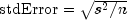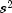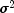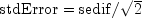JMSLTM Numerical Library 7.2.0
com.imsl.stat

## Class MultipleComparisons

• All Implemented Interfaces:
Serializable, Cloneable

```public class MultipleComparisons
extends Object
implements Serializable, Cloneable```
Performs Student-Newman-Keuls multiple comparisons test.

Class `MultipleComparisons` performs a multiple comparison analysis of means using the Student-Newman-Keuls method. The null hypothesis is equality of all possible ordered subsets of a set of means. This null hypothesis is tested using the Studentized range of each of the corresponding subsets of sample means. The method is discussed in many elementary statistics texts, e.g., Kirk (1982, pp. 123-125).

Example, Serialized Form
• ### Constructor Summary

Constructors
Constructor and Description
```MultipleComparisons(double[] means, int df, double stdError)```
Constructor for `MultipleComparisons`.
• ### Method Summary

Methods
Modifier and Type Method and Description
`int[]` `compute()`
Performs Student-Newman-Keuls multiple comparisons test.
`void` `setAlpha(double alpha)`
Sets the significance level of the test
• ### Methods inherited from class java.lang.Object

`clone, equals, finalize, getClass, hashCode, notify, notifyAll, toString, wait, wait, wait`
• ### Constructor Detail

• #### MultipleComparisons

```public MultipleComparisons(double[] means,
int df,
double stdError)```
Constructor for `MultipleComparisons`.
Parameters:
`means` - A `double` array containing the means.
`df` - An `int` scalar containing the degrees of freedom associated with `stdError`.
`stdError` - A `double` scalar containing the effective estimated standard error of a mean. In fixed effects models, `stdError` equals the estimated standard error of a mean. For example, in a one-way modelwhereis the estimate ofand n is the number of responses in a sample mean. In models with random components, usewhere `sedif` is the estimated standard error of the difference of two means.
• ### Method Detail

• #### compute

`public final int[] compute()`
Performs Student-Newman-Keuls multiple comparisons test.
Returns:
An `int` array , call it `equalMeans` indicating the size of the groups of means declared to be equal. Value `equalMeans[I] = J` indicates the I-th smallest mean and the next J-1 larger means are declared equal. Value `equalMeans[I] = 0` indicates no group of means starts with the I-th smallest mean.
• #### setAlpha

`public void setAlpha(double alpha)`
Sets the significance level of the test
Parameters:
`alpha` - A `double` scalar containing the significance level of test. `alpha` must be in the interval [0.01, 0.10]. Default: alpha = 0.01
JMSLTM Numerical Library 7.2.0